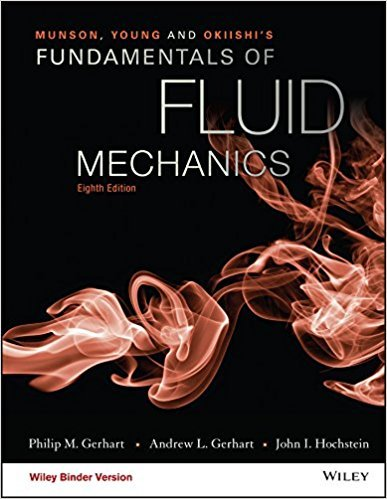×
×

# One end of a pond has a shoreline that resembles a halfbodyas shown in Fig. P6.55. AISBN: 9781119080701 456

## Solution for problem 6.55 Chapter 6.6

Fundamentals of Fluid Mechanics | 8th Edition

• Textbook Solutions
• 2901 Step-by-step solutions solved by professors and subject experts
• Get 24/7 help from StudySoup virtual teaching assistantsFundamentals of Fluid Mechanics | 8th Edition

4 5 1 372 Reviews
10
1
Problem 6.55

One end of a pond has a shoreline that resembles a halfbodyas shown in Fig. P6.55. A vertical porous pipe is locatednear the end of the pond so that water can be pumped out. Whenwater is pumped at the rate of 0.08 m3/s through a 3-m-long pipe,what will be the velocity at point A? Hint: Consider the flow insidea half-body. (See Video V6.5.)

Step-by-Step Solution:
Step 1 of 3

12/3/2014 6:58 PM Wave Interference and Diffraction Reflection and Interference from Thin Films ▯ Normal-incidence light strikes surface covered by...

Step 2 of 3

Step 3 of 3

##### ISBN: 9781119080701

This full solution covers the following key subjects: . This expansive textbook survival guide covers 112 chapters, and 1357 solutions. Since the solution to 6.55 from 6.6 chapter was answered, more than 259 students have viewed the full step-by-step answer. The answer to “One end of a pond has a shoreline that resembles a halfbodyas shown in Fig. P6.55. A vertical porous pipe is locatednear the end of the pond so that water can be pumped out. Whenwater is pumped at the rate of 0.08 m3/s through a 3-m-long pipe,what will be the velocity at point A? Hint: Consider the flow insidea half-body. (See Video V6.5.)” is broken down into a number of easy to follow steps, and 63 words. This textbook survival guide was created for the textbook: Fundamentals of Fluid Mechanics, edition: 8. Fundamentals of Fluid Mechanics was written by and is associated to the ISBN: 9781119080701. The full step-by-step solution to problem: 6.55 from chapter: 6.6 was answered by , our top Science solution expert on 03/16/18, 03:21PM.

Unlock Textbook Solution